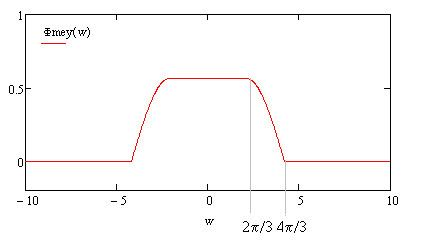# Meyer wavelet

Updated onThe Meyer wavelet is an orthogonal wavelet proposed by Yves Meyer. It is infinitely differentiable with infinite support and defined in frequency domain in terms of function ν as:

Ψ ( ω ) := { 1 2 π sin ( π 2 ν ( 3 | ω | 2 π 1 ) ) e j ω / 2 if  2 π / 3 < | ω | < 4 π / 3 , 1 2 π cos ( π 2 ν ( 3 | ω | 4 π 1 ) ) e j ω / 2 if  4 π / 3 < | ω | < 8 π / 3 , 0 otherwise ,

where:

ν ( x ) := { 0 if  x < 0 , x if  0 < x < 1 , 1 if  x > 1.

There are many different ways for defining this auxiliary function, which yields variants of the Meyer wavelet. For instance, another standard implementation adopts

ν ( x ) := { x 4 ( 35 84 x + 70 x 2 20 x 3 ) if  0 < x < 1 , 0 otherwise .

The Meyer scale function is given by:

Φ ( ω ) := { 1 2 π if  | ω | < 2 π / 3 , 1 2 π cos ( π 2 ν ( 3 | ω | 2 π 1 ) ) if  2 π / 3 < | ω | < 4 π / 3 , 0 otherwise .

In the time-domain, the waveform of the Meyer mother-wavelet has the shape as shown in the following figure:

In 2015, Victor Vermehren Valenzuela and H. M. de Oliveira gave the explicitly expressions of Meyer wavelet and scale functions:

ϕ ( t ) = { 2 3 + 4 3 π t = 0 , sin ( 2 π 3 t ) + 4 3 t cos ( 4 π 3 t ) π t 16 π 9 t 3 o t h e r w i s e ,

and

ψ ( t ) = ψ 1 ( t ) + ψ 2 ( t )

where

ψ 1 ( t ) = 4 3 π ( t 1 2 ) cos [ 2 π 3 ( t 1 2 ) ] 1 π sin [ 4 π 3 ( t 1 2 ) ] ( t 1 2 ) 16 9 ( t 1 2 ) 3 ,

and

ψ 2 ( t ) = 8 3 π ( t 1 2 ) cos [ 8 π 3 ( t 1 2 ) ] + 1 π sin [ 4 π 3 ( t 1 2 ) ] ( t 1 2 ) 64 9 ( t 1 2 ) 3 .

Similar Topics
Seema Bowri
Victor Parsonnet